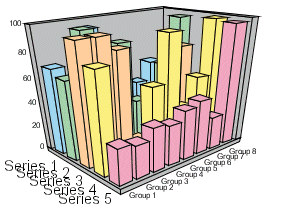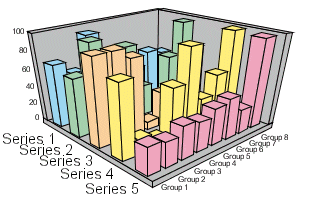CubeProportional

When a 3D graph type is selected, this property tells the chart to define the axis size proportional to the number of series or groups.

Syntax:

`setCubeProportional(boolean);`
`boolean = getCubeProportional();`

where:

boolean

Can be one of the following:

true draws cube proportional to number of groups. This value is the default.

false draws cube proportional to number of series.

Example:

`setGraphType(0);  setCubeProportional(true);``setGraphType(0);  setCubeProportional(false);`WebFOCUS